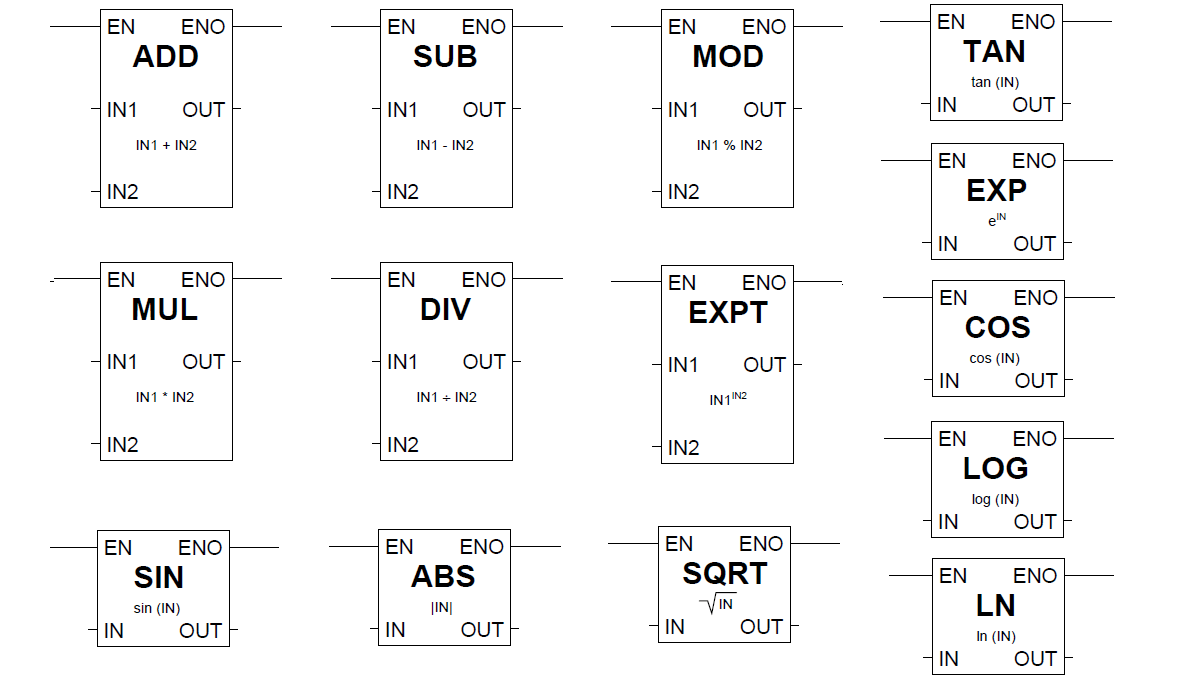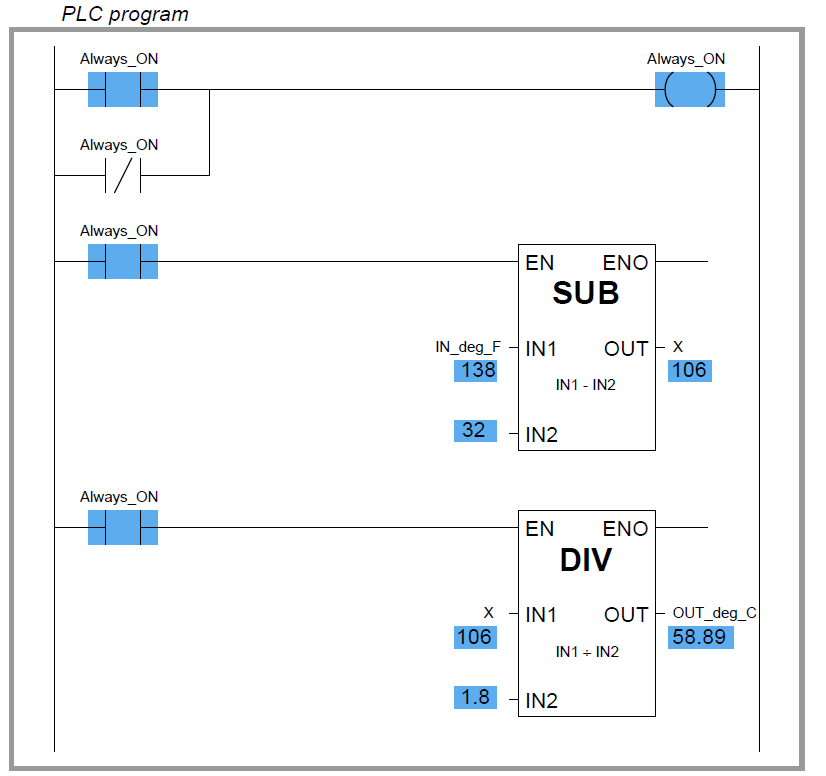Home » PLC Math instructions
PLC Tutorials

# PLC Math instructions

The IEC 61131-3 standard specifies several dedicated ladder instructions for performing arithmetic calculations. Some of them are shown here:

## PLC Math instructionsAs with the data comparison instructions, each of these math instructions must be enabled by an “energized” signal to the enable (EN) input. Input and output values are linked to each math instruction by tag name.

An example showing the use of such instructions is shown here, converting a temperature measurement in units of degrees Fahrenheit to units of degrees Celsius.

In this particular case, the program inputs a temperature measurement of 138 deg F and calculates the equivalent temperature of 58.89 deg C:well as a dedicated variable (X) used to store the intermediate calculation between the subtraction and the division “boxes.”

Although not specified in the IEC 61131-3 standard, many programmable logic controllers support Ladder Diagram math instructions allowing the direct entry of arbitrary equations.

Rockwell (Allen-Bradley) Logix5000 programming, for example, has the “Compute” (CPT) function, which allows any typed expression to be computed in a single instruction as opposed to using several dedicated math instructions such as “Add,” “Subtract,” etc.

General-purpose math instructions dramatically shorten the length of a ladder program compared to the use of dedicated math instructions for any applications requiring non-trivial calculations.

For example, the same Fahrenheit-to-Celsius temperature conversion program implemented in Logix5000 programming only requires a single math instruction and no declarations of intermediate variables:Credits : by Tony R. Kuphaldt – Creative Commons Attribution 4.0 License

#### Troubleshoot Allen Bradley PLC Logic

This website uses cookies to improve your experience. We'll assume you're ok with this, but you can opt-out if you wish. Accept Read More

WordPress Image Lightbox

Send this to a friend